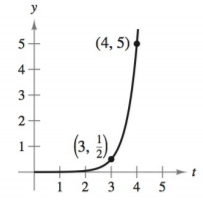Chapter 4.6, Problem 6E### Calculus: An Applied Approach (Min...

10th Edition
Ron Larson
ISBN: 9781305860919

#### Solutions

Chapter
Section### Calculus: An Applied Approach (Min...

10th Edition
Ron Larson
ISBN: 9781305860919
Textbook Problem
1 views

# Modeling Exponential Growth and Decay In Exercises 1-6, find the exponential function y = C e k t that passes through the two given points. y = C e k tTo determine

The exponential function y=Cekt for the following graph:Explanation

Given Information:

The provided graph is:

Consider the following graph:

Observe that the exponential function curve passes through the points (3,12) and (4,5).

Hence the points (3,12) and (4,5) satisfy the equation of exponential function y=Cekt.

Substitute t=3,y=12 in the equation y=Cekt.

12=Cek(3)12=Ce3k

Substitute t=4,y=5 in the equation y=Cekt.

5=Cek(4)5=Ce4k

Now take the ratio of the equations Ce3k=12 and Ce4k=5,

Ce4kCe3k=512e4ke3k=25e4k3k=10ek=10

Take natural logarithm of both the sides

### Still sussing out bartleby?

Check out a sample textbook solution.

See a sample solution

#### The Solution to Your Study Problems

Bartleby provides explanations to thousands of textbook problems written by our experts, many with advanced degrees!

Get Started

#### Place the following set of n = 20 scores in a frequency distribution table.

Essentials of Statistics for The Behavioral Sciences (MindTap Course List)

#### perform the indicated operations and simplify each expression. 127. (x2 + 2)2 [5(x2 + 2)2 3](2x)

Applied Calculus for the Managerial, Life, and Social Sciences: A Brief Approach

#### In problems 1-16, solve each equation. 10. Solve

Mathematical Applications for the Management, Life, and Social Sciences

#### Evaluate: 12x21xdx. a) 322 b) 2+21 c) 4+2 d) 14

Study Guide for Stewart's Single Variable Calculus: Early Transcendentals, 8th